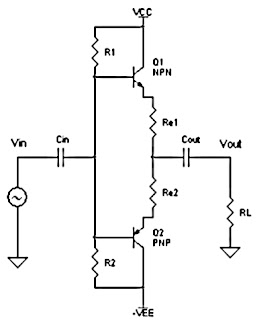# Class B power amplifier circuit

Excessive heat to be problematic in the amplifier Class A. Then be made to class B amplifier with a point Q is shifted to point B. Point B is a point on the line load ,where this point intersects the line current Ib = 0. Because of the location of point Such, then the transistor works only active in one part wave phase only.

Therefore class B amplifier is always made with 2 of the transistor Q1 (NPN) and Q2 (PNP).Point Q amplifier A, AB and B

Since both these transistors work alternately, then the amplifier class B is often named as Push-Pull amplifier. If the signal a sine wave, then transistor Q1 is active in 50% of cycles The first (positive phase 0o-180o) and The next turn on transistor Q2 50% in the next cycle (phase negative 180o - 360o). Class B amplifier more efficient than the class A, because if there is no input signal (vin = 0 volts) then the bias current also Ib = 0 and practically made ​​a second trasistor is turned OFF.Series Class B Power Amplifier
Class B amplifier efficiency approximately by 75%. But that does not mean the problem is over, because the transistor have to-not-an ideal. In in fact there kirakira VBE voltage clamp of 0.7 volts which causes transistor is in OFF state although the flow has a larger Ib some mA from 0. This is what led to the emergence of crossover problems active transition at the time of the transistor Q1 to the transistor Q2 turns become active.